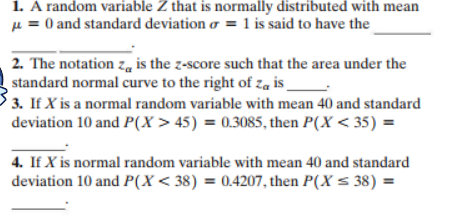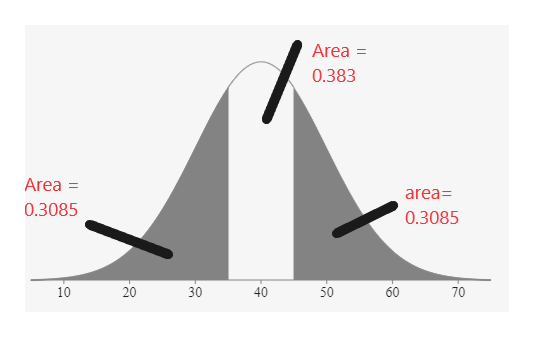# 1. A random variable Z that is normally distributed with mean0 and standard deviation o = 1 is said to have the2. The notation z is the z-score such that the area under thestandard normal curve to the right of za is3. IfX is a normal random variable with mean 40 and standarddeviation 10 and P(X> 45) = 0.3085, then P(X< 35)4. If X is normal random variable with mean 40 and standarddeviation 10 and P(X < 38) = 0.4207, then P(X= 38) =

Question
99 views

question 1-4help_outlineImage Transcriptionclose1. A random variable Z that is normally distributed with mean 0 and standard deviation o = 1 is said to have the 2. The notation z is the z-score such that the area under the standard normal curve to the right of za is 3. IfX is a normal random variable with mean 40 and standard deviation 10 and P(X> 45) = 0.3085, then P(X< 35) 4. If X is normal random variable with mean 40 and standard deviation 10 and P(X < 38) = 0.4207, then P(X= 38) = fullscreen
check_circle

Step 1

A standard normal distribution is normally distributed with mean µ = 0 and standard deviation σ = 1

1)

A random variable Z that is normally distributed with mean µ = 0 and standard deviation σ =  is said to have the Standard Normal Distribution

2) The notation Zα is the z-score such that the area under the standard normal curve to the right of Zα  is 1- α

Here total area is 1, area to the left is α

So, Total area to the right is 1- α

3)

As per the question

Mean = 40

Standard deviation = 10

P(X>45) = 0.3085

P(X>40) = 0.5

P(40<X<45)= 0.5 – 0.3085 = 0.1915

P(X<40) = 0.5

P(35<X<40) = P(40<X<45) = 0.1915 (Because Normal distribution table is symmetrically distributed)

P(X<35) = P(X<40)- P(35<X<40) = 0.5 – 0.1915 = 0.3085

It is clearly shown in the table belowhelp_outlineImage TranscriptioncloseArea 0.383 Area = area 0.3085 0.3085 10 20 30 40 50 60 70 fullscreen
Step 2

4)

In continuous rando...

### Want to see the full answer?

See Solution

#### Want to see this answer and more?

Solutions are written by subject experts who are available 24/7. Questions are typically answered within 1 hour.*

See Solution
*Response times may vary by subject and question.
Tagged in

### Other